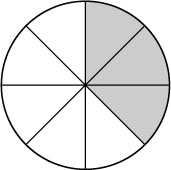Numbers, units and arithmetic

This free course is available to start right now. Review the full course description and key learning outcomes and create an account and enrol if you want a free statement of participation.

Free course

# 1.7 Fractions

A fraction is written as one number over another (such as) and means the top number divided by the bottom number. The top number, 3, is called the numerator and the bottom number, 10, is called the denominator. Whole numbers can be written as fractions with the denominator 1. Thus, 2 can be written as. The same fraction may be written in different ways, depending upon the context.

The top of a fraction is called the numerator.

The bottom of a fraction is called the denominator.

Click on the tabs below and note that all of the shaded areas represent the same fraction of the circle: one third of it. SoActive content not displayed. This content requires JavaScript to be enabled.
Interactive feature not available in single page view (see it in standard view).

Fractions which are equal in value but are written with different numerators and denominators are called equivalent fractions.

You can find equivalent fractions for any given fraction by multiplying top and bottom by the same whole number.

Decimals are themselves a special form of fractions (0.3 is). There are situations where it is simpler to use fractions. For example, if you wanted to divide a circle into six equal pieces, you think of the fraction. There is no exact decimal equivalent of(because dividing 6 into 1.0000 … produces a never-ending string of 6s: 0.1666 …). Recipes often ask for fractions, such as ‘half a pint of milk’ or a cooking time of ‘three-quarters of an hour’.

## Example 5

What fraction of the circle is shaded in the diagram below?The fraction is. The circle is divided into 8 equal sections, so each section is. Three of them are shaded so the shaded part isof the circle.

However there are other equivalent fractions which represent the same shaded area, obtained by multipying the numerator and the denominator by the same whole number, e.g..

MU120_4M1